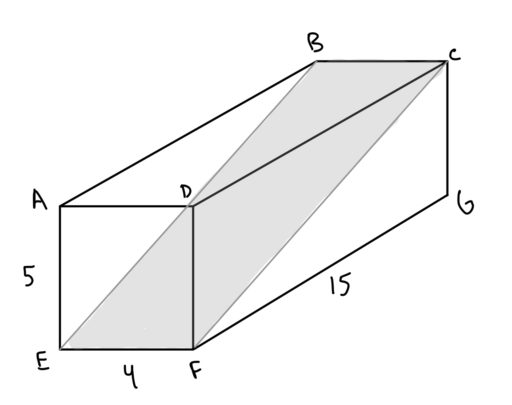One important kind of 3D shape is the rectangular solid: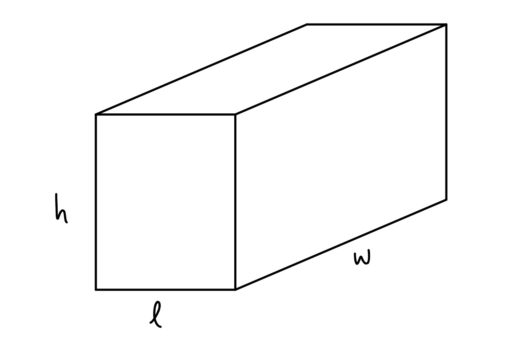which is defined as follows:

Rectangular Solid: A 3D shape with six faces which are all rectangles placed perpendicularly to one another.

The fact that the faces are all perpendicular to each other helps a great deal in calculating the volume, for we simply have:

Volume of a Rectangular Solid: For the following rectangular solid,its volume is equal toExample 1
Find the area of a rectangular solid whose dimensions are.

Example 2
A rectangular solid has two sides that are 3 and 8 units long. It has a volume of 48 units. What is the length of its remaining side?

And, to find the surface area of any rectangular solid, we simply need to add up the area of each of the 6 rectangles which surround it.

Surface Area of a Rectangular Solid: For the following rectangular solid,its surface area is equal toHow did we get this formula? Well, since each of the faces are perpendicular to each other, we know that there are two rectangles with sides ofandunits long, one in front and one at the back: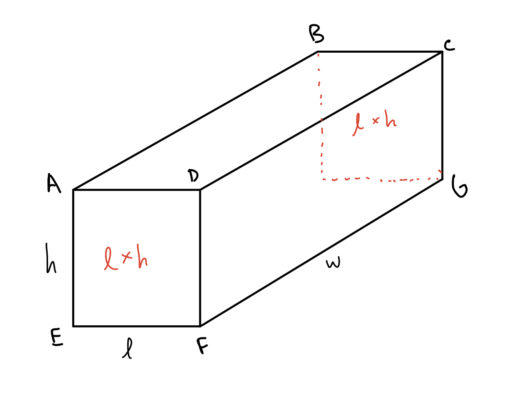and there are two with sides ofunits long: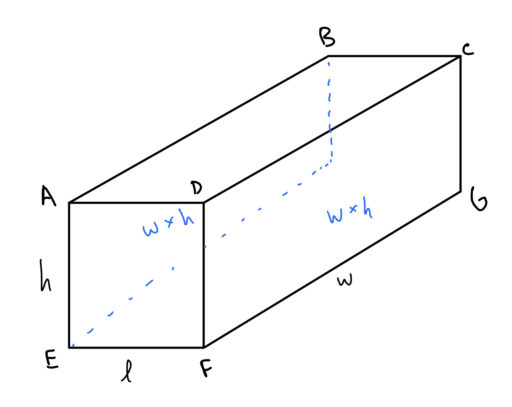and there are two with sides ofunits long: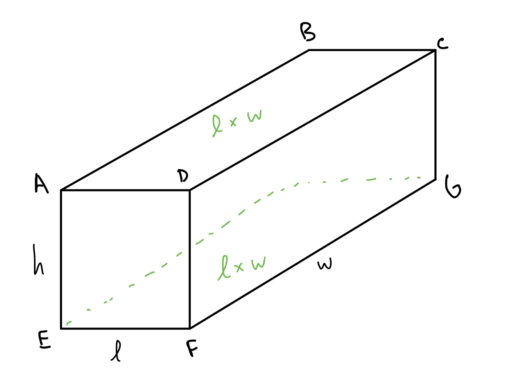Thus, adding up the area of all of those rectangles, we get:which is the same as what we wrote above.

Now, one common 3D shape is the cube, and the cube is just a special kind of rectangular solid: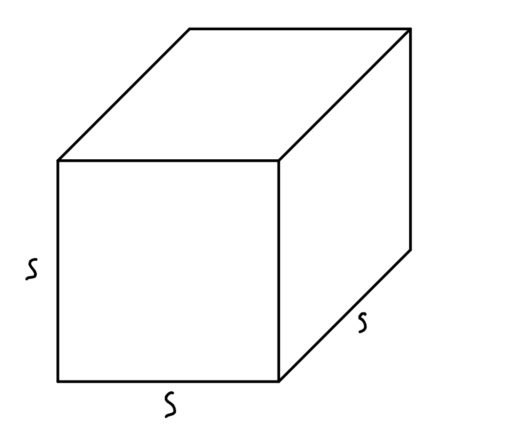Cube: a cube is a rectangular solid whose six sides are all squares.

Our formulas become especially simple for a cube. Letbe the length of any side of the cube:

Volume of a Cube: The volume of a cube is.

Surface Area of a Cube: The surface area of a cube is.

These formulas follow directly from just applying our above formulas for the volume/surface area of any rectangular solid.

Finally, it is worth noting how we can use the Pythagorean Theorem to find certain distances on a rectangular solid. Consider the following problem:

Example 3
For a rectangular solid with a length of, a width ofand a height of, find the length of: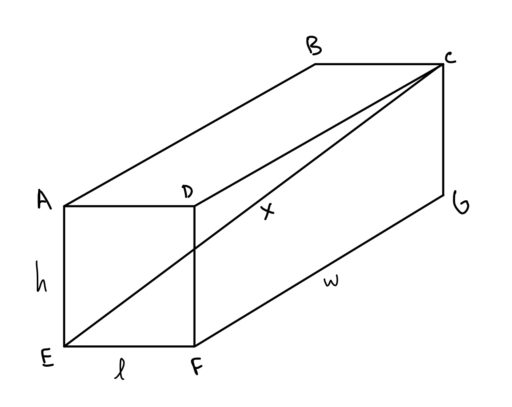Practice Problems

1. Find the length of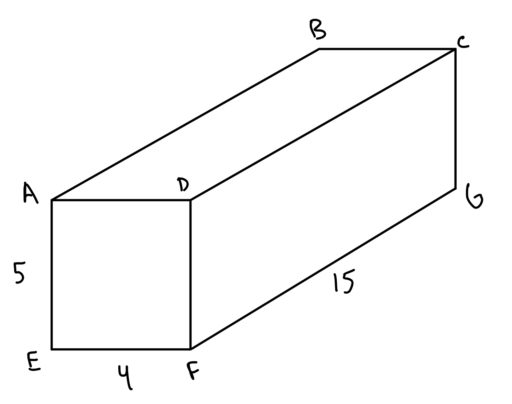2. Find the length of.3. Find the area of the shaded planebelow: